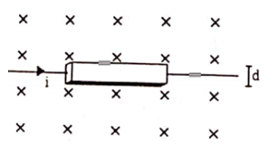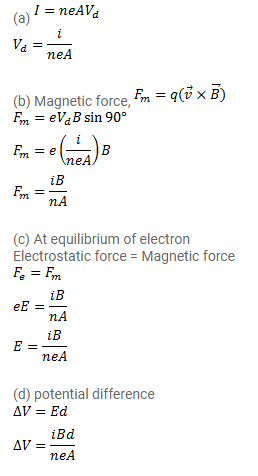# A current i is passed through a silver strip of width d and width d and area of cross-section A.

Question:

A current $i$ is passed through a silver strip of width $d$ and width $d$ and area of cross-section $A$. The number of free electrons per unit volume is $\mathrm{n}$.

(a) Find the drift velocity $\mathrm{v}$ of the electrons.

(b) If a magnetic field $B$ exists in the region as shown in figure, what is the average magnetic force on the free electrons?

(c) Due to the magnetic force, the free electrons get accumulated on one side of the conductor along its length. This produces a transverse electric field in the conductor which opposes the magnetic force on the electrons. Find the magnitude of the electric field which will stop further accumulation of electrons.

(d) What will be the potential difference developed across the width of the conductor due to the electronaccumulation? The appearance of a transverse emf, when a current-carrying wire is placed in a magnetic field, is called Hall effect.Solution: